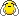# How to do a histogram from a data file

Hello there,

Sorry for posting this question, which could already be answered, buy I really could not find the way to look for it inside the Forum.
I’m a beginner, so this can be a naive question. I have been trying by reading the User Manual, but I really don’t understand, so I hope someone can give me a hand.
So, what I need to know is how I can generate a Histogram, with some data I have stored in a file. To be more specific, in the file, I have written the following information, related to an energy spectrum:

Center-of-the-bin, Bin-width, Bin(bar)-Height, bError[/b].

There are 11 bins.
I have written the following ‘code’ trying to get the histogram:

``````void plot_histo(){
//------------- Style --------------
gROOT->SetStyle("Plain");
gStyle->SetOptStat(0);
//------------- Style --------------

TH1D *h1 = new TH1D("h1","",10,0.2,3.0);
double ene = 0;
double bin = 0;
double dat = 0;
double err = 0;
int cont = 0;

double x1,x2;
double y1,y2;
double w1,w2;

ifstream datafile("datarelease0509/histogram1.txt"); //calling the data file
TMatrixD dArray(11,3);

for(int i = 0 ; i < 11 ; i++)
for(int j = 0 ; j < 3 ; j++)
datafile >> dArray(i,j);

if( datafile.is_open() ){
do {
cont++;
datafile >> ene >> bin >> dat;

x1 = ene/1000.0;
y1 = dat/bin;
w1 = bin;

h1->Fill(x1,y1);
printf("%3d Ene= %f  Dat=%f  \n",cont,x1,y1);

}while (!datafile.eof());
}//-if datafile.is_open

TCanvas *C = new TCanvas("C","C", 100, 100);

C->cd();
h1->Draw("");

C->Print("hist_1.eps");
}``````

I would appreciate if you can help me here.Cheers,

Mario AAO.

Hi,

this works:

``````void plot_histo(){
//------------- Style --------------
gROOT->SetStyle("Plain");
gStyle->SetOptStat(0);
//------------- Style --------------

ifstream datafile("d.txt"); //calling the data file
double x, w, c, e, bins;

for (int i = 0 ; i < 11; i++) {
datafile >> x[i] >> w[i] >> c[i] >> e[i];
double upper = x[i] + w[i] / 2.;
double lower = x[i] - w[i] / 2.;
bins[i + 1] = upper;
printf("bin[%d] = %g\n", i + 1, upper);
if (i == 0) {
bins[i] = lower;
printf("bin[%d] = %g\n", i, lower);
} else if (fabs(bins[i] - lower) > 0.01) {
Error("Bin setup",
"Lower bin edge %g for bin %d contradicts upper bin edge %g for bin %d!",
lower, i, bins[i], i - 1);
}
}

TH1D *h1 = new TH1D("h1","",11, bins);

for(int i = 0 ; i < 11 ; i++) {
int bin = h1->GetXaxis()->FindFixBin(x[i]);
h1->SetBinContent(bin,c[i]);
h1->SetBinError(bin, e[i]);
}

TCanvas *C = new TCanvas("C","C", 100, 100);

C->cd();
h1->Draw("");

}``````

Cheers, Axel.